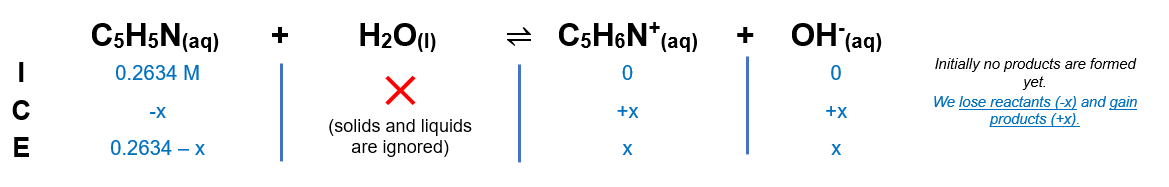# Problem: Pyridine is a weak base with the formula, C5H5N, the Kb for Pyridine is 1.8 x 10-9 In an aqueous solution, pyridine partially dissociates according to the following reaction: C5H5N + H2O ⇔ C5H5NH+ + OH-Use the Kb equation to calculate the pH of the aqueous pyridine solution described below: Volume: 375 mL Concentration: 0.2634 M Since this is a weak base, you can assume the amount of base dissociated is &lt;&lt; 5% of the total amount of base present. Report your answer with the correct number of significant digits.

###### FREE Expert Solution

We’re being asked to calculate the pH of a 0.2634 M C5H5N

C5H5N is a weak base that will further react in water to form OH-

C5H5N(aq) + H2O (l) → OH- (aq) + C5H6N+ (aq)

From this, we can construct an ICE table for the reaction of C5H5N in a solution. Remember that liquids are ignored in the ICE table.97% (22 ratings)###### Problem Details

Pyridine is a weak base with the formula, C5H5N, the Kb for Pyridine is 1.8 x 10-9 In an aqueous solution, pyridine partially dissociates according to the following reaction: C5H5N + H2O ⇔ C5H5NH+ + OH-

Use the Kb equation to calculate the pH of the aqueous pyridine solution described below: Volume: 375 mL Concentration: 0.2634 M Since this is a weak base, you can assume the amount of base dissociated is << 5% of the total amount of base present. Report your answer with the correct number of significant digits.